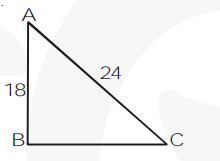# A guy wire attached to a vertical pole of height 18 m is 24 m long and has a stake attached to the other end.

Question. A guy wire attached to a vertical pole of height 18 m is 24 m long and has a stake attachedto the other end. How far from the base of the pole should the stake be driven so that the wirewill be taut?
Solution:

Let AB be the vertical pole of 18 m and AC be the wire of 24 m.

The $\Delta \mathrm{ABC}$, by pythagoras theorem$\mathrm{AC}^{2}=\mathrm{AB}^{2}+\mathrm{BC}^{2}$

$24^{2}=18^{2}+\mathrm{BC}^{2}$

$\mathrm{BC}^{2}=252$

$\mathrm{BC}=6 \sqrt{7} \mathrm{~m}$